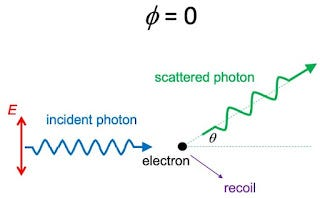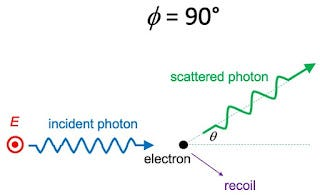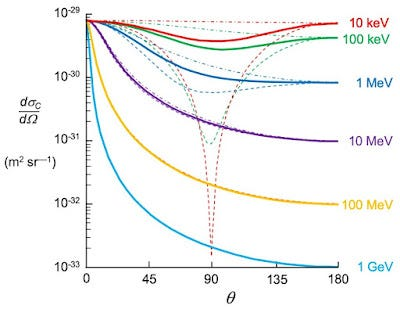Klein-Nishina Formula for Polarized Photons

In Chapter 15 of Intermediate Physics for Medicine and Biology, Russ Hobbie and I discuss Compton Scattering. An incident photon scatters off a free electron, producing a scattered photon and a recoiling electron. We write

The inclusion of dynamics, which allows us to determine the relative number of photons scattered at each angle, is fairly complicated. The quantum-mechanical result is known as the Klein-Nishina formula (Attix 1986). The result depends on the polarization of the photons. For unpolarized photons, the cross section per unit solid angle for a photon to be scattered at angle θ is

where

is the classical radius of the electron. [The variable x is the ratio of the incident photon’s energy to the rest energy of the electron.]

What happens for polarized photons? In that case, the scattering may depend on the angle φ with respect to the direction of the electric field. The resulting scattering formula is

Unpolarized light means that you average over all angles φ, implying that factors of cos^2φ become ½. A bit of algebra should convince you that when the expression above is averaged over φ it’s equivalent to Eq. 15.16 in IPMB.

In order to analyze polarized photons, we must consider the two polarization states, φ = 0 and φ = 90°.

The incident and scattered photon directions define a plane. Assume the electric field associated with the incident photon lies in this plane, as shown in the drawing below. From a classical point of view, the electric field will cause the electron to oscillate, resulting in dipole radiation (a process called Thomson scattering). A dipole radiates perpendicular to its direction of oscillation, but not parallel to it. Therefore, you get scattering for θ = 0 and 180°, but not for θ = 90°.A schematic diagram of Compton scattering for polarized light with φ = 0.

A quantum-mechanical analysis of this behavior (Compton scattering) accounts for the momentum of the incident photon and the recoil of the electron. In the quantum case, some scattering occurs at θ = 90°, but it is suppressed unless the energy of the incident photon is much greater than the rest mass of the electron ( x >> 1).

For Thomson scattering, if the electric field oscillates perpendicular to the scattering plane (shown below) then all angles θ are perpendicular to the dipole and therefore should radiate equally. This effect is also evident in a quantum analysis unless x >> 1.A schematic diagram of Compton scattering for polarized light with φ = 90°.

The figure below is similar to Fig. 15.6 in IPMB. The thick, solid lines indicate the amount of scattering (the differential cross section) for unpolarized light, as functions of θ. The thin dashed curves show the scattering for φ = 0 and the thin dash-dot curves show it for φ = 90°. The red curves are for a 10 keV photon, whose energy is much less than the 511 keV rest energy of an electron ( x << 1). The behavior is close to that of Thomson scattering. The light blue curves are for a 1 GeV photon (1,000,000 keV). For such a high energy ( x >> 1) almost all the energy goes to the recoiling electron, with little to the scattered photon. The dashed and dash-dot curves are present, but they overlap with the solid curve and are not distinguishable from it. Polarization makes little difference at high energies.The differential cross section for Compton scattering. The incident photon energy for each curve is shown on the right. The solid curves are for unpolarized light, the dashed curves are for light with φ = 0, and the dash-dot curves are for φ = 90°. Adapted from Fig. 15.6 in IPMB.

Why is there so little backscattering (θ = 180°) for high energy photons? It’s because the photon has too much momentum to have its direction reversed by a light electron. It would be like a truck colliding with a mosquito, and after the collision the truck recoils backwards. That’s extraordinarily unlikely. We all know what will happen: the truck will barrel on through with little change to its direction. Any scattering occurs at small angles.

Notice that Thomson scattering treats light as a wave and predicts what an oscillating electric field will do to an electron. Compton scattering treats light as a photon having energy and momentum, which interacts with an electron like two colliding billiard balls. That is wave-particle duality, which is at the heart of a quantum view of the world. Who says IPMB doesn’t do quantum mechanics?

Originally published at http://hobbieroth.blogspot.com.